Courses

# Surveying (Part - 13) Civil Engineering (CE) Notes | EduRev

## Civil Engineering (CE) : Surveying (Part - 13) Civil Engineering (CE) Notes | EduRev

The document Surveying (Part - 13) Civil Engineering (CE) Notes | EduRev is a part of the Civil Engineering (CE) Course Civil Engineering SSC JE (Technical).
All you need of Civil Engineering (CE) at this link: Civil Engineering (CE)

Triangulations computation

(1) Checking the means of the observed angles
(2) Checking the triangular errors
(3) Checking the total round of each station
(4) Computation of the length of the base line
(5) Computation of the sides of the main triangle
(6) Satellite computation if any.
(7) Sag correction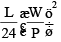where, W = Total weight of the tape L = Horizontal distance between

Always subtractive

(8) Reduction to M.S.L. The difference in the legnth at sea-level, is known as error due to reduction to M.S.L.
Correction due to reduction to M.S.L.
L= L - L'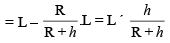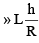Reconnance Preliminary field inspection of the  entire area to be concerned by triangulation is known as reconsamce.
It includes the following operations:

(i) Proper examination of the terrian
(ii) Selection of suitable  position for base lines
(iii) Selection of suitable position of triangulation stations.
(iv) Determination of intervisibility of triangulation stations.

Determination of intervisibility of stations It there is no obstruction due to intervening ground, the distance of visible horizontal from the station of known elevation above mean sea level, may be calculated with the formula. h = 0.06735D2

• While deciding the intervisibility of various stations, the line of sight should be at least 3 metres above the point of tangency of the earth’s surface to avoid grazing sights
• Intervisibility os tations intervened by ground profiles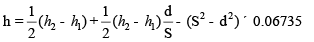Atmospheric calibaration of instruments A typical set of standard conditions used in calibrating an infrared instruments of wave length 0.875 mm are pressure of 760 mm of mercury and a temperature of 12ºC. The value of standardising index (ns) is obtained as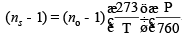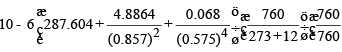nS=1.000282

Determination of correct distances If a line is measure with an E.D.M instruments under atmospheric conditions which are not the same as the standard conditions, the measured distance will be incorrect. The correct distance (D) is obtained from the relation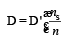where D' = recorded distance
ns = standarding refractive index, and
n = refractive inded at the time of measurement

Slop and Height Correction Slop correction,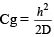where h = elevation differences between the two ends.
Height correction,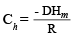where Hm = mean elevation of the instrument station.
Effect of Atmospheric conditions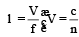when measuring distance with an E.D.M. instrument, the effect of atmospheric on the wave length should be considered and a suitable correction is applied.
In E.D.M. instruments, the distance in measured in terms of the wave length, where as in correction in taping, it is measured in terms of the tape length.
Determination of n (a) For instruments using carrier waves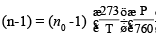where P = atmospheric pressure in mm of Hg.
T = absolute temperature in degree kelvin = 273 + Tº and n= refractive index ratio of air at 0ºC and 760 mm of Hg.
The value of n0 is obtained as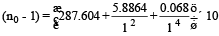where l is the wave length of the carrier wave in mm.
The above equation are applicable to the E.D.M. instruments using visible light, laser and enforced waves.

(b) For instruments using microwave

.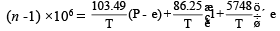where t1 is the total time from A to B and back to A at the transmitter end.
At the receiver end, the reverse process of demodulation occurs in which the meaning wave is separated from the carrier wave and the phase difference of the meaning wave is determined.
The carrier waves used in E.D.M. instrument are basically of two forms

(1) Visible or infrared light waves
(2) Microwaves

Method of Modulation The meaning wave is superimposed on the carrier wave and this process in called modulation. The following two methods of modulation are used, depending upon the nature of carrier wave.

(i) Amplitude modulation (A.M.) or intensity modulation
(ii) Frequency modulation (F.M)

(i) Amplitude modulation . Amlitude modulation is used when light waves are the carrier waves. In this method, the amplitude of the carrier waves is varied in direct proportion to the amplitude of the measuring wave (modulating wave). However the frequency remains costant.

(ii) Frequency modulation . Frequency modulations in used in microwave instruments. In frequency modulation, the frequency, of the carrier wave is varied in proportion to the frequency of the measuring wave, However the amplitude of the carrier wave remains constant.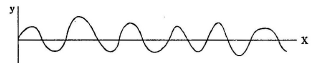In general, 2D =nl + Dl

or D = (1/2) (nl + 4a)

where n = number of whole waves tranvelled by the wave from A to b and back from B to A Dl = fraction of the wave length travelled by the wave from A to b and back from B to A The value of Dl depends upon the phase difference of the wave transmitted and that received back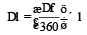where Df = phase difference

Carrier Waves The electromagnetic waves whole wave lengths are suitable for measuring the distance have the frequency in the range of 500m Hz to 7.5 MHz. This corresponds to the wave lengths in the rnge of 0.6 to 40m. These waves are called measuring waves. The measuring waves are also known as modulation waves.

Types of waves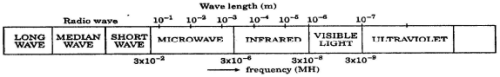Distance from measurement of transit time Let us consider a survey line AB whose length is to be measured with an E.D.M. instrument. the unit at A is a transmitter which propagets an electromagnetic wave in the direction towards the receiver B.
When the wave is generated at A, an electronic time starts at B1 and as soon as the wave reaches B, the timer stay. Thus the transit time of the wave from A to b is determined. This distance (D) travelled from A to B can be found as where D = V × T V = velocity of the curve t = transit time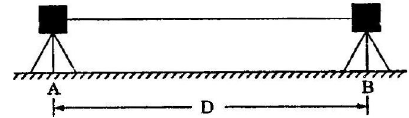When the unit at A acts both as a transmitter and a receiver,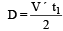Phase of the wave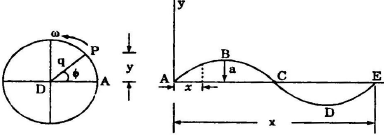We assumed that the vector OP is at the initial line when t = 0 i.e., f = 0.
Let us assume that the wave starts from the positionA. it sweeps out on angle f = wt with respect to the initial line OA in time.
The displacement y along the y-axis is given by y = a sin f = a sin (wt) ...............(i)

The displacement x along the x-axis is given by x = Vt ...............(ii)

From equation (i) and (ii)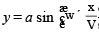..............(iii)

We know that equation of the electromagnetic wave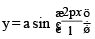........... (iv)

Let AB is a survey line. The wave is transmitted from the unit at A towards B. It is instantly reflected by the reflector at B and then received back to A. Fig (a) and (c) shows the details of the electromagnetic wave from A to b and back from B to A From A to B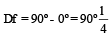............. (i)

Again from B to A,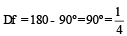\ Distance between B and A = D = 2l+ l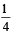......(ii)

The phase difference between the wave at A when transmitted and when received back is Df -180o - 0o = 180o = 1/2. Unfortunately an electromagnetic wave of the frequency range 500 MHz is to 7.5 MHz to not suitable for direct transmission through the atmosphere. This is due to the effect of interference, reflection, fading and scatter, overcome the difficulty of direct transmission, the measuring wave is electronically superimposed on one wave called the carrier wave. The carrier wave is of much high frequency.
Infrared E.D.M instrument In infrared E.D.M. instruments the near infrared radiation bond of wave length 0.9 x 10"° is used as a carrier wave. As the carrier were length is close to the visible light spectrum, the infrared instruments are sometimes classified in electro-optical instruments.
This type of E.D.M. instrument can be used upto range limited to 2 to 3 km. The accuracy is usually ±10 mm

Microwave E.D.M. Instruments

• The Tellurometer was the first instrument which used microwave in the measurement of distance for surveying purposes.
• The carrier wave frequencies used in the microwave E.D.M. instruments are in the range of 3 x 103 MHz to 3 x 104 MHz (X = 0.1 m to 0.01 m). These waves can be transmitted over long distances upto 100 km.

The carrier waves are frequency modulated.
The Tellurometer system consists of two identical units, called the master instrument and the remote instrument. As the units are indentical each unit can be switched to operate either of a master unit or remote unit. (This is unlike a geodimeter which has only one instrument) but function is same.

The range of the microwave E.D.M. instruments is generally between 30 to 80 km. The accuracy is of the order of ± 15 mm plus 5mm/km. These instruments are mainly for establishing control for very large projects.

Electro optical E.D.M. instruments or light wave E.D.M. instruments

The electro optical E.D.M. instruments use lightwaves as carrier waves. The instruments was called geodimeter.

The geodimeter transmits a light beam of wave length 0.55 x 10-6 m (f= 5.5 x 108 MHz) to which a modulation of about 30 MHz (l = 10 m) is applied. The light wave is amplitude modulated. The light beam is directed on to a reflector at the other end of the line which is to be measured. The reflector reflects back the light beam to the instruments. The incoming light beam is received by a photo electric cell which measures the difference between the transmitted and received modulation.

The geodimeter consists of the following 5 main components
(i) Light source
(ii) Electro-optical shutter
(iii) Oscillator or modulation generator
(iv) Transmitting and receiving optical system.
(v) Photo-electric cell.

Working of a Geodimeter : A continuous waves carrier beam of light is generated in the transmitter. It is modulated by an electro-optical shutter. It then enters the transmitting optical system and is transmitted to the far end of the line. It is reflected back to the instruments by the reflector. It is received by the receiving optical system and relayed to the photo cell detector for phase comparison.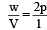or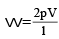As one revolution is completed in time T, we have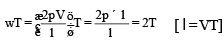Thus when the radial vector OP completes one revolution the wave travels through a distane equal to the wave length (l).
Measurement of distance from phase difference The phase difference is equal to the difference of the phase angle of the reflected signal and the phase angle of the transmitted signal. Thus

Df = f-f1
where f2 = Phase difference
f1 = Phase angle of the transmitted signal
f2 = Phase angle of the reflected signal

Offer running on EduRev: Apply code STAYHOME200 to get INR 200 off on our premium plan EduRev Infinity!

## Civil Engineering SSC JE (Technical)

109 docs|50 tests

,

,

,

,

,

,

,

,

,

,

,

,

,

,

,

,

,

,

,

,

,

;